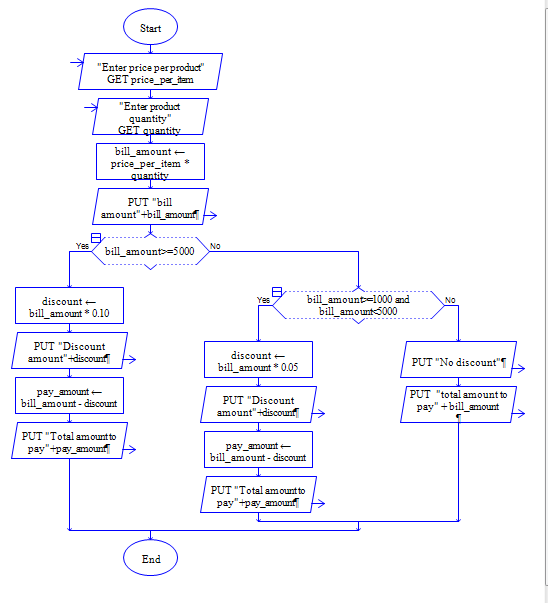# Java Program to Calculate Different Discounts to Bill Amount

Get price and quantity of a product, calculate bill amount, calculate 10% discount for the bill amount 5000 and above, 5% discount for the bill amount 1000 and above.

Sample Input 1:

10 400

Sample Output 1:

3800

Sample Input 2:

10 500

Sample Output 2:

4500

#### Flow Chart Design#### Program or Solution

``` //To Calculate Different Discount For Different Bill Amount... import java.util.*; class Program { public static void main(String args[]) { int qua,price; double amount=0,discount=0; Scanner sc=new Scanner(System.in); System.out.println("Enter The Price:"); price=sc.nextInt(); System.out.println("Enter The Quantity:"); qua=sc.nextInt(); amount=price*qua; if(amount>=5000) { discount=amount*0.10; } else if(amount>1000) { discount=amount*0.05; } else { discount=0; } amount=amount-discount; System.out.println("The Amount Is:"+amount); } } ```